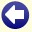## We Prove To You:      All Our Puzzles Are Interesting!

Let S be the set of uninteresting puzzles on our site:

Assume that S is not empty:
This means that there are some uninteresting puzzles on our site.
Then there also exists one least interesting puzzle.
Let P (member of S) be this least interesting puzzle:
Then we would be very interested to know which puzzle P is.
This makes P a very interesting puzzle!
However, we assumed that P was uninteresting (member of S).back to the main puzzle page